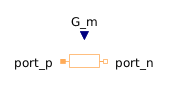Wrap Angle - MapleSim Help

Wrap Angle

Wrap angleDescription The Wrap Angle component wraps the input angle into the half-open interval $\left(-\mathrm{\pi },\mathrm{\pi }\right]$, if the parameter Positive range is false. Otherwise the input angle is wrapped to the interval $\left[0,2\mathrm{\pi }\right)$.EquationsConnections

 Name Description Modelica ID $u$ Real input signal u $y$ Real output signal yParameters

 Name Default Units Description Modelica ID Positive range $\mathrm{false}$ True (checked) mean output only positive values positiveRangeModelica Standard Library The component described in this topic is from the Modelica Standard Library. To view the original documentation, which includes author and copyright information, click here.